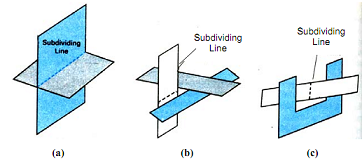## Explain the scan-line algorithm, Data Structure & Algorithms

Assignment Help:

Explain the Scan-Line Algorithm

This image-space method for removing hidden surfaces is an extension of the scan-line algorithm for filling polygon interiors. Instead of filling just one surface, we now deal with multiple surfaces. As each scan line is processed, all polygon surfaces intersecting that line are examined to determine which are visible. Across each scan line, depth calculations are made for each overlapping surface to determine which is nearest to the view plane. When the visible surface has been determined, the intensity value for that position is entered into the refresh buffer.

We assume that tables are set up for the various surfaces, which include both an edge table and a polygon table. The edge table contains coordinate end points for each line in the scene, the inverse slope of each line, and pointers into the polygon table to identify the surfaces bounded by each line. The polygon table contains coefficients of the plane equation for each surface, intensity information for the surfaces, and possibly pointers into the edge table. To facilitate the search for surfaces crossing a given scan line, we can set up an active list of edges from information in the edge table. This active list will contain only edges that cross the current scan line, sorted in order of increasing x. In addition, we define a flag for each surface that is set on or off to indicate whether a position along a scan line is inside or outside of the surface. Scan lines are processed from left to right. At the leftmost boundary of a surface, the surface flag is turned on; and at the rightmost boundary, it is turned off.  Figure 3.6 illustrates the scan-line method for locating visible portions of surface for pixel positions along the line. The active list for scan line 1 contains information from the edge table for edges AB and BC, only the flag for surface S1 is on. Therefore, no depth calculations are necessary, and intensity information for surface S1 is entered from the polygon table into the refresh buffer. Similarly, between edges EH and FG, only the flag for surface S2 is on. No other positions along scan line 1 intersect surfaces, so the intensity values in the other areas are set to the background intensity. The background \intensity can be loaded throughout the buffer in an initialization routine.

For scan lines 2 and 3 in Figure, the active edge list contains edges AD, EH, BC, and FG. Along scan line 2 from edge AD to edge EH, only the flag for surface S1 is on. But between edges EH and BC, the flags for both surfaces are on. In this interval, depth calculations must be made using the plane coefficients for the two surfaces. For this example, the depth of surface S1 is assumed to be less than that of S2, so intensities for surface S1 are loaded into the refresh buffer until boundary BC is encountered. Then the flag for surface S1 goes off, and intensities for surface S2, so intensities for surface S1 are loaded into the refresh buffer until boundary BC is encountered. Then the flag for surface S1 goes off, and intensities for surface S2 are stored until edge FG is passed.

We can take advantage of coherence along the scan lines as we pass from one scan line to the next. In Figure 3.6, scan line 3 has the same active list of edges as scan line 2. Since no changes have occurred in line intersections, it is unnecessary again to make depth calculations between edges EH and BC. The two surfaces must be in the same orientation as determined on scan line 2, so the intensities for surface S1 can be entered without further calculations.#### Binary search, In a sorted list, Binary search is carried out by dividing t...

In a sorted list, Binary search is carried out by dividing the list into two parts depends on the comparison of the key. Since the search interval halves each time, the iteration o

#### Algorithm for stack using array, write an algorithm for stack using array p...

write an algorithm for stack using array performing the operations as insertion ,deletion , display,isempty,isfull.

#### Flow chart, What is tha flow chart of algorithm

What is tha flow chart of algorithm

#### Registers, what are registers? why we need register? Definition? Types? Wha...

what are registers? why we need register? Definition? Types? What registers can do for us?

#### Row major storage, Q. Take an array A[20, 10] of your own. Suppose 4 words ...

Q. Take an array A[20, 10] of your own. Suppose 4 words per memory cell and the base address of array A is 100. Find the address of A[11, 5] supposed row major storage.

#### Recursion, difference between recursion and iteration

difference between recursion and iteration

#### Binary search tree, Objectives The purpose of this project is to give yo...

Objectives The purpose of this project is to give you significant exposure to Binary Search Trees (BST), tree traversals, and recursive code. Background An arbitrary BST i

#### 2 Flow Charts, 1.Create a flowchart to show the process that will allow the...

1.Create a flowchart to show the process that will allow the implementation of Stack, Push, and Pop operations. 2.Create a flowchart to show the process that will allow the impleme

#### Representation of data structure in memory, Representation of data structur...

Representation of data structure in memory is known as: Abstract data type

#### Algorithmss, calculate gpa using an algorithm

calculate gpa using an algorithm